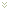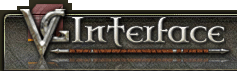Search Downloads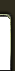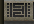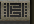User Name:

Remember Me?
##### Views

Authors: Grimmier

In Part 1 of this tutorial we learned about UseControls and StaticWindows, and how to use them to add graphics to your window. Now we are going to use that knowledge to create a mini experience window.

• Lets fist open up the Testing.xml file we created in Part 1.
• Go down to the bottom of the page and delete the </XML> tag.
• Add in a blank line, we are going to just add our next window to the same xml file. (XML Files can hold many windows at a time, the VGUIWindow tag will mark where each one begins and ends)
• Lets add in a comment so we can find and fix any problems we have later on down the line. Add in the line <!-- EXP Window --> the <!-- and --> tags on the end mark everything inside as a comment, telling the engine not to try and read that line.

We will begin with the same blank window we created in the Creating the Window lesson. Add the window after the comment, Change the window name to "Testing XP" It should look like this.

```Code:
<!-- EXP Window -->
<VGUIWindow name="Testing XP">
<Rect x="400" y="200" width="200" height="100"/>
<Settings  visible="true"
border="frameall"
background="true"
movable="true">
</Settings>
</VGUIWindow>
</XML>
```

Since we want to make this into a smaller window, lets go with a smaller sized fill bar. For this exercise I decided to go with the standard HP/END/ENG bar style. The masks for this are located in the Shells/Default/Textures/VgrdParts.xml file.

Code:

```      <AnimationInfo name="PlayerGreenMeterFill">
<AnimationFrame tick="1000">
<TextureName>VgrdParts.tga</TextureName>
<Rect x="606" y="114" width="155" height="9" />
<HotSpot>
<Point x="0" y="0" />
</HotSpot>
<Offset>
<Point x="0" y="0" />
</Offset>
</AnimationFrame>
</AnimationInfo>
```

I went with the Green one =).

Now remember the 3 important things to note.

1. Name PlayerGreenMeterFill
• NOTE remember that the name is actually a 2 part process. that is the 2nd half of the name, the first is Vgrd which can be found at the top of the file.
Making the Full Name VgrdPlayerGreenMeterFill
2. width 155
3. height 9

Now that we know the 3 important parts of the image, lets see how to add one to the window. I borrowed this code from the END Bar on the default player window.

Code:

```		<UseControl	type="StatusBar"
<StatusBar emptyGraphic="BarEmpty"
fullGraphic="BarFull">
<Rect x="76" y="47" width="156" height="9"/>
<GraphicName label="BarEmpty" isBaseName="false">
<Name>VgrdPlayerGreenMeterFillEmpty</Name>
</GraphicName>
<GraphicName label="BarFull" isBaseName="false">
<Name>VgrdPlayerGreenMeterFill</Name>
</GraphicName>
</StatusBar>
<Settings
name="_status_endurance"
notifyOnLeftClick="true"
notifyOnRightClick="true"/>
</UseControl>
```

Starting at the top, You can see we are going to be using a UseControl again.

Notice the type this time is StatusBar

There is another attribute as well, gameVarLink this attribute tells the engine that whatever the output of this control is, is linked directly to a value contained inside a game variable.

• Since we do not want to see the END bar again and want this one to show us EXP, lets replace this with the Adventuring Experiance gameVarLink of "AdvExpPctSelf" there is also ones for crafting XP and harvesting XP, as well as Debt.
For now we won't worry about debt just positive XP.

Instead of using a StaticWindow this time to act as a frame for our image its a StatusBar (ala the type of UseControl).

• a Status bar much like a StaticWindow has a few attributes you can set.
In this case its one for the emptyGraphic and one for fullGraphic
Used to tell the engine what image to show for each isntance.
There is one masked for empty in the VgrdParts.xml file as well you can check if you want =)

The next part we should recognize it sets the location and dimentions of the object we are adding.

• Notice how the width and height are about the same size as the fill bar we looked at earlier.
• You want your frame to always be the same size or larger, the image will not be rescaled to fit.

Now this part is exactly the same as adding in any other image to a window you just have 2 images this time, Empty and Full.

• I would like to remind everyone that the game engine will read your XML files from top to bottom, it will also draw the images in the order it reads them.

After you add the 2 images for the bar, you can close the StatusBar tag.

We don't need to set up any additional settings for this so we can skip that part and close the UseControl tag.

Lets set our location for the status bar to be 10 x 10 away from the top left corner, to give it a little buffer.

Code:

```<!-- EXP Window -->
<VGUIWindow name="Testing XP">
<Rect x="400" y="200" width="200" height="100"/>
<Settings  visible="true"
border="frameall"
background="true"
movable="true">
</Settings>
<UseControl	type="StatusBar"
<StatusBar emptyGraphic="BarEmpty"
fullGraphic="BarFull">
<Rect x="10" y="10" width="156" height="9"/>
<GraphicName label="BarEmpty" isBaseName="false">
<Name>VgrdPlayerGreenMeterFillEmpty</Name>
</GraphicName>
<GraphicName label="BarFull" isBaseName="false">
<Name>VgrdPlayerGreenMeterFill</Name>
</GraphicName>
</StatusBar>
</UseControl>
```

Now our window will have a bar on it that show our XP bar in a smaller window with green fill.

Lets add the % values to the right end of our bar now.

To do this we will need to use another UseControl, this time the type is TextField, very similar to the other 2 UseControls we have already used.

• First you start with the UseControl tag and tell it the type "TextField"
• Next since we are going to be linking our text to the value of a game variable, we need a gameVarLink in this case "AdvExpPctSelf" the same as the bar.
• Status Bars use a percentage variable to know how far to move the bars, when you link this value to text you will get a fraction. ::To get around this you add the "Percent" formatting suffix to the end like this "AdvExpPctSelf.Percent".

Code:

```		<UseControl	type="TextField"
```
• Now we can add in the TextField, there are quite a few attributes you can set for a text field, settings like font type, color, size etc. for now we are only going to work with size, color, and centered.
• Lets stick with something easy to see for now, size of 10 and white text, and centered to false.
• Setting centered to false will force align left format, this will make it easier to line up our text.

Code:

```<TextField fontSize="10" color="white" centered="false">
```
• Next we set the location since its only moving to the right and not up or down we need to change the X value.
X will be the starting X + the width so 176, lets put a visual buffer of 4 pixels and make it an even 170.
• The width and height you set the text field, depends on how many characters you plan to fit and the size of the font.
As a general rule of thumb think of your font size as being a box with the width being 2 pixels larger than its size, and the height being 2x the size, so for fontSize ="10" think of 12x20 boxes. That is the size of one character. Since the max percent is 100 thats 3 characters, the width would be 12x3 = 36
• To keep bar centered on the text lets raise the text up by 5.

Code:

```<Rect x="180" y="5" width="36" height="20"/>
```

• Now comes the actual text we want displayed, this is denoted by <Text></Text> tags, with whats inside being displayed on screen.
Since we are linking this text field to a gameVar we can leave this blank, since whatever the gamevar is will overwrite what we put in once the variables are loaded into memory.

• Now we can close our the TextField tags and the UseControl as well.

Code:

```<UseControl	type="TextField"
<TextField fontSize="10" color="white" centered="false">
<Rect x="180" y="5" width="36" height="20"/>
<Text></Text>
</TextField>
</UseControl>
```
• This method here will not add in the % sign at the end of the value, you will only see the number displayed.
You can either add this manually and tweak the layout to get it exact or there are some inline Text Formatting tricks you can use, more on that in the Advanced section.

• Now we can close the Window and XML tags so the whole page should look like this.

Code:

```   <VGUIWindow name="Testing XP">
<Rect x="400" y="200" width="200" height="100"/>
<Settings  visible="true"
border="frameall"
background="true"
movable="true">
</Settings>
<UseControl	type="StatusBar"
<StatusBar emptyGraphic="BarEmpty"
fullGraphic="BarFull">
<Rect x="10" y="10" width="156" height="9"/>
<GraphicName label="BarEmpty" isBaseName="false">
<Name>VgrdPlayerGreenMeterFillEmpty</Name>
</GraphicName>
<GraphicName label="BarFull" isBaseName="false">
<Name>VgrdPlayerGreenMeterFill</Name>
</GraphicName>
</StatusBar>
</UseControl>
<UseControl	type="TextField"
<TextField fontSize="10" color="white" centered="false">
<Rect x="180" y="5" width="36" height="20"/>
<Text></Text>
</TextField>
</UseControl>
</VGUIWindow>
</XML>
```

You will notice if you look that the total window size is to tall and not wide enough for our bar and text display.

• Lets correct that, our total width of the window should be at least to the end ot the last item (the text field). Lets give it a buffer of 10 as well to kind of center it. so 176 + 36 = 212 + 10 = 222.
• The height to be centered should be start location + height of tallest item + buffer. 10 + 20 + 10 = 40. + the frame all border will eat up some of our window, about 5 pixels in all directions, lets add another 10. so 50.

Here is what the entire Testing.xml should look like

Code:

```<?xml version="1.0" standalone="yes"?>
<XML id="SGOUIMarkupLanguage" version="0.1"
xmlns="http://www.sigilgames.com/vanguard-ui-0.1"
xmlns:xsi="http://www.w3.org/2001/XMLSchema-instance"
xsi:schemaLocation="http://www.sigilgames.com/vanguard-ui-0.1 ../../../vanguard-ui.xsd">
<VGUIWindow name="Testing">
<Rect x="200" y="400" width="200" height="100"/>
<Settings  visible="true"
border="frameall"
background="true"
movable="true">
</Settings>
<UseControl	type="StaticWindow">
<StaticWindow pictureMode="true" textData="ClassGraphic">
<Rect x="0" y="0" width="65" height="81"/>
<GraphicName	label="ClassGraphic" isBaseName="false">
<Name>VgrdClassRogue</Name>
</GraphicName>
</StaticWindow>
<Settings tooltip="Rogue"
visible="true"/>
</UseControl>
<UseControl	type="StaticWindow">
<StaticWindow pictureMode="true" textData="ClassGraphic">
<Rect x="65" y="5" width="65" height="81"/>
<GraphicName	label="ClassGraphic" isBaseName="false">
<Name>VgrdClassCleric</Name>
</GraphicName>
</StaticWindow>
<Settings tooltip="Cleric"
visible="true"/>
</UseControl>
<UseControl	type="StaticWindow">
<StaticWindow pictureMode="true" textData="ClassGraphic">
<Rect x="130" y="10" width="65" height="81"/>
<GraphicName	label="ClassGraphic" isBaseName="false">
<Name>VgrdClassSorcerer</Name>
</GraphicName>
</StaticWindow>
<Settings tooltip="Sorcerer"
visible="true"/>
</UseControl>
</VGUIWindow>
<!-- EXP Window -->
<VGUIWindow name="Testing XP">
<Rect x="400" y="200" width="222" height="50"/>
<Settings  visible="true"
border="frameall"
background="true"
movable="true">
</Settings>
<UseControl	type="StatusBar"
<StatusBar emptyGraphic="BarEmpty"
fullGraphic="BarFull">
<Rect x="10" y="10" width="156" height="9"/>
<GraphicName label="BarEmpty" isBaseName="false">
<Name>VgrdPlayerGreenMeterFillEmpty</Name>
</GraphicName>
<GraphicName label="BarFull" isBaseName="false">
<Name>VgrdPlayerGreenMeterFill</Name>
</GraphicName>
</StatusBar>
</UseControl>
<UseControl	type="TextField"
<TextField fontSize="10" color="white" centered="false">
<Rect x="180" y="5" width="36" height="20"/>
<Text></Text>
</TextField>
</UseControl>
</VGUIWindow>
</XML>
```

Check back for Part 3...Our Network